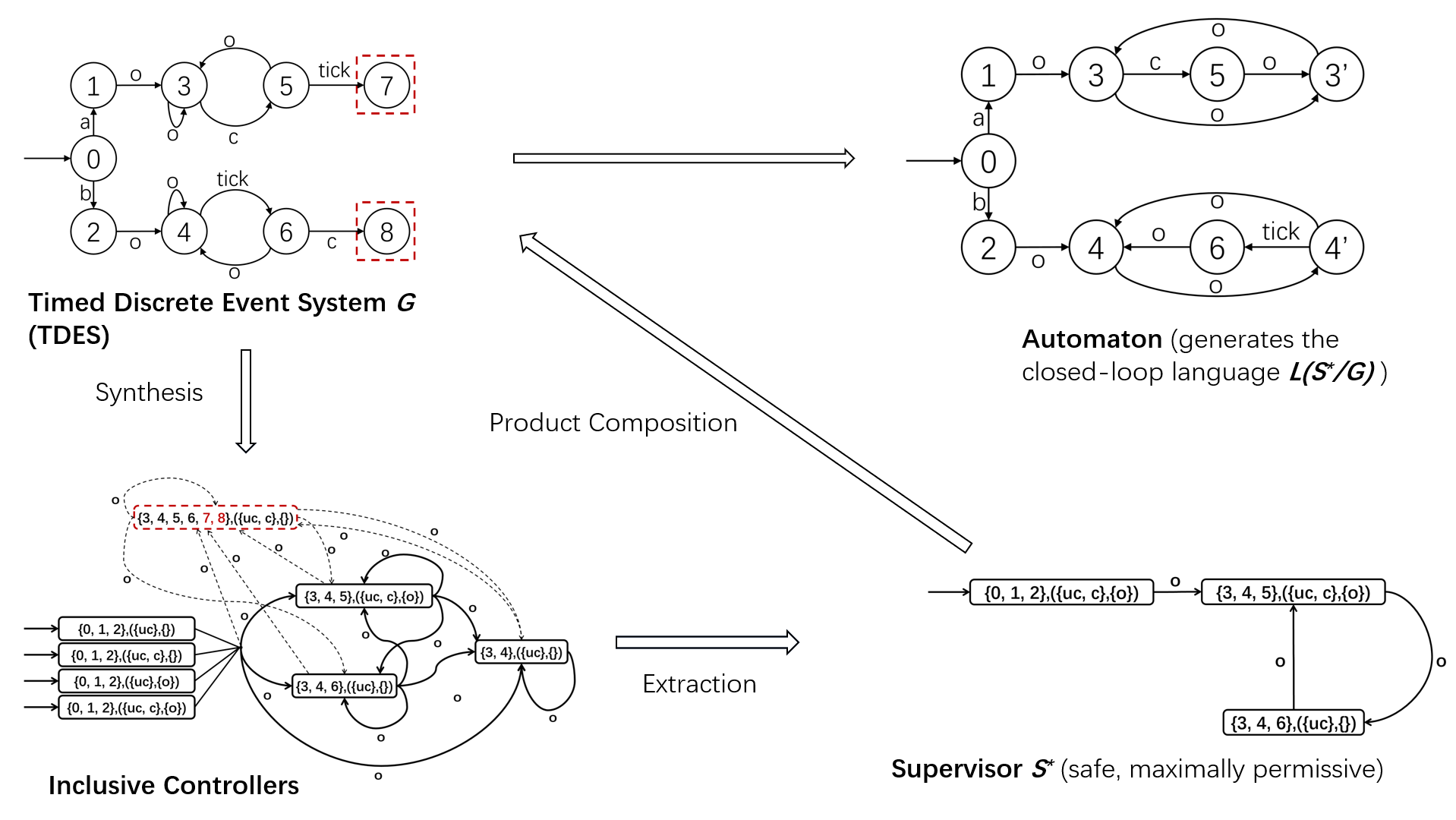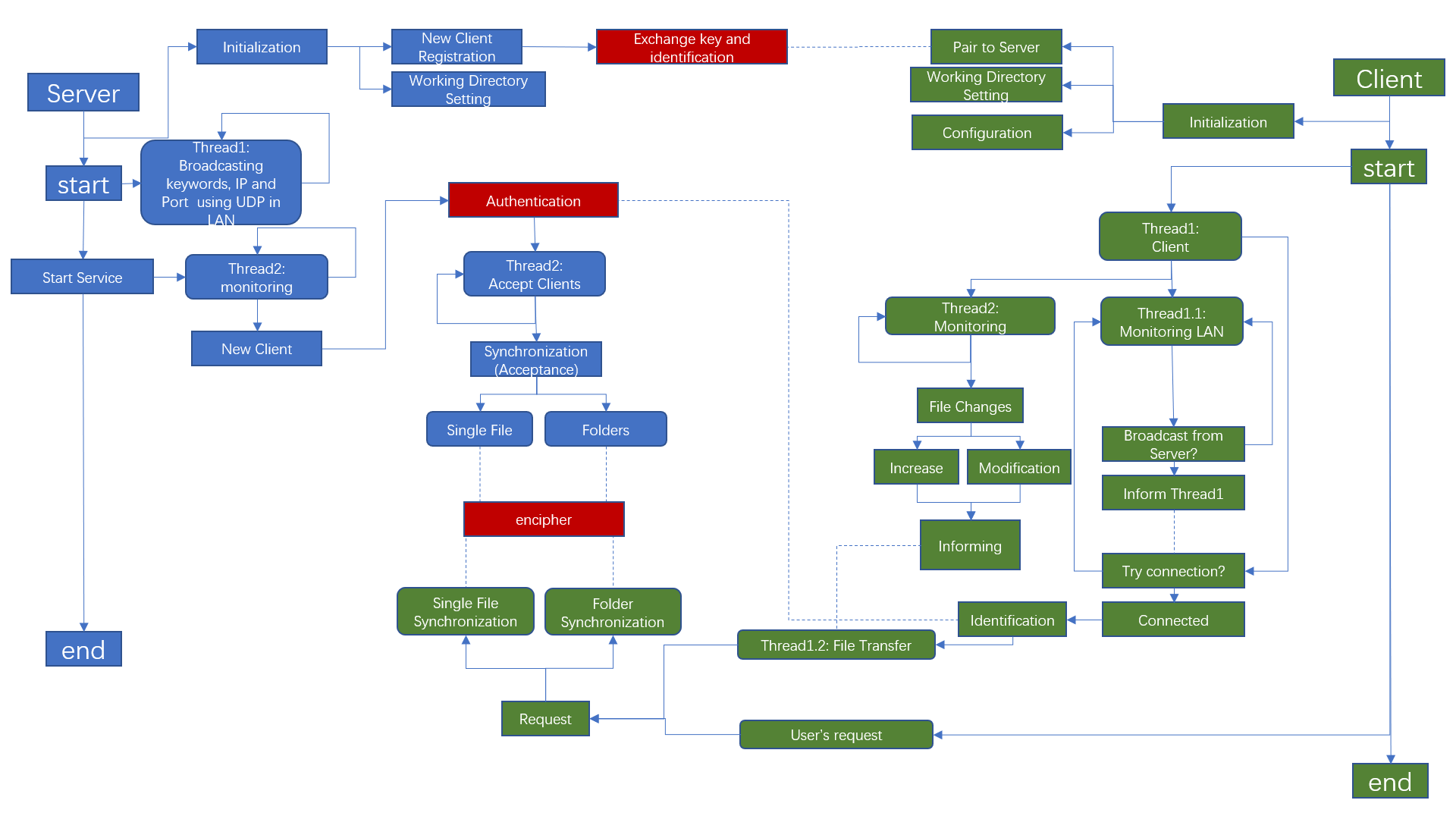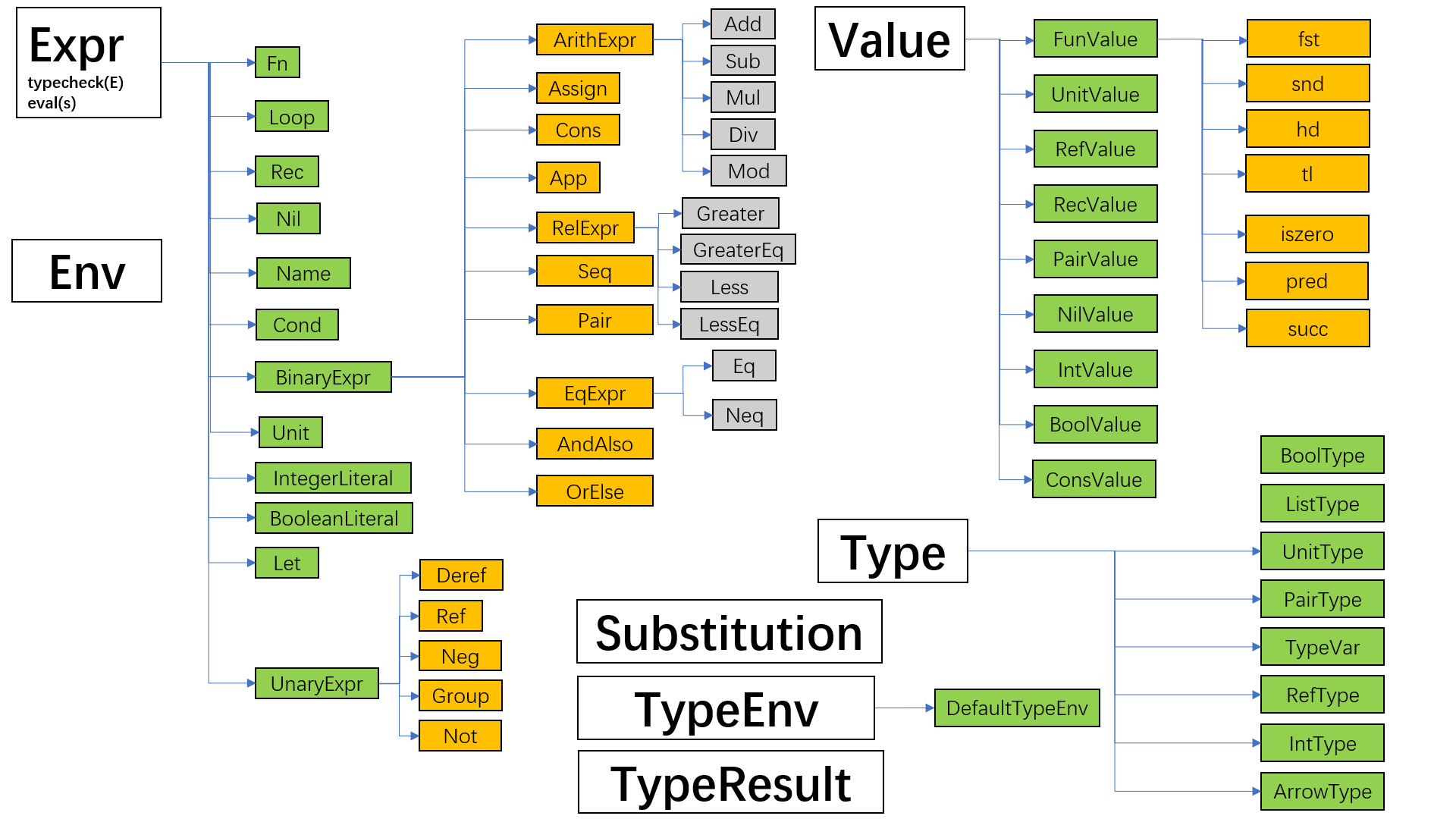# Research Projects

## Verification-aided Compiler Optimization

[Note: This project was now continued by Hanzhi Liu, an undergraduate genius student (2019~2023, expected) and an experienced programmer at SJTU, also advised by Qinxiang Cao, to implement this optimization and framework in VST’s separation logic [VST = Verified Software Toolchain]]

We are designing a new method of program optimization for C programs with assertion annotated that contains verification information of the program. The new methods was being realized based on original CompCert compiler. New semantics framework was designed based on CompCert small step semantic framework. A conditional definition of “forward simulation” and “backward simulation” diagram for compiler correctness was proposed with crucial properties proved.

This is an original work of our research group under exploration, so details are still not opened to the public.

## Finite Canonical Model for Completeness Theory in Coq Based on UnifySL:

UnifySL is a library of the proof assistant Coq designed for basic mathematical logic (e.g. Propositional Logics, Separation Logics etc.) under development (not open sourced for now). Formalized proof theories, semantic definitions, soundness proofs and completeness proofs were abstractly described using Coq’s type classes and high-order features. The completeness proof of previous logics are all based on the framework of infinite canonical model.

My work as a research assistant aimed to investigate the finite model methods, starting the exploration from the formalizing of propositional dynamic logic (PDL), including the proof theories, semantic definitions, the proof of its soundness and the proof of its weak completeness, making the best of framework and tools in UnifySL. Currently the completeness proof was finished, and we expect to extract a new framework of high-order abstraction for finite model methods in the future.

During my work, a lot of useful lemmas was also proved as a supplementary of the UnifySL library.

Implementation Details

The following presented several representative and critical definitions and lemmas:

• The abstract type class of PDL:

``````Class Program : Type:={
program: Type;
}.

Class PropositionalDynamicLanguage (L:Language)(Pro: Program):Type:={
boxp: program -> expr -> expr
}.

Class ProgramOperation (L:Language)(Pro:Program):Type:= {
choice: program -> program -> program;
composition: program -> program -> program;
iteration: program -> program;
test: expr -> program
}.
``````

Note: we can describe Classical PDL abstractly by composing the type classes above of classical logic. The same applies to the proof system and semantic system.

• The deep-embedded syntax of PDL

``````Inductive program {Sigma: PropositionalVariables}{ProV: ProgramVariables}: Type :=
| choice: program -> program -> program
| composition: program -> program -> program
| iteration: program -> program
| test: expr -> program
| basep: BaseP -> program
with
expr {Sigma: PropositionalVariables} {ProV: ProgramVariables}: Type :=
| impp : expr -> expr -> expr
| orp : expr -> expr -> expr
| andp: expr -> expr -> expr
| falsep : expr
| boxp: program -> expr -> expr
| varp : Var -> expr.
``````

Note: we must indicate explicitly in Coq that the given syntax is an instance of the abstract type class. The same applies to the proof system and semantic system.

• The Truth Lemma and Existence Lemma (mutually inductive):

``````Lemma TRUTH_LEMMA:
forall (psi x: exp) m Phi,
rel psi m Phi ->
FL_closure_construction psi x ->
(KRIPKE: canonical_Kmodel psi, m |= x <-> proj1_sig Phi x)
with EXISTENCE_LEMMA:
forall (pi: prog)(psi x: exp)(m : Kworlds (canonical_Kmodel psi)),
FL_Atom psi (proj1_sig m) ->
FL_closure_construction psi ([pi]x) ->
(proj1_sig m ([pi]x) <-> forall n, R_D pi m n -> proj1_sig n x).
``````

Note: these are two core lemmas for proving completeness of modal logic and its extension.

• The Weak Completeness Theorem

``````  (**
The general abstract definition of weak completeness property:
Definition weakly_complete {L: Language} (Gamma: Provable L) {MD: Model} (SM: Semantics L MD) (MC: ModelClass MD): Prop :=
forall x: expr, valid MC x -> provable x.
**)
Theorem complete_weakly: weakly_complete GP SM (KripkeModelClass _ kMC).
``````

## Supervisor Control of Timed Discrete-Event Systems

The supervisory control theory of Discrete Event Systems (DES) is a formal framework for the synthesis of control logic for complex automated systems. We investigated the supervisory control problem for timed discrete-event systems (TDES) under partial observation where time was considered as a special event. The design objective is to synthesize a maximally-permissive supervisor to restrict the behavior of the system such that the closed-loop language is within a safe specification language. Relevant paper was accepted by 21st IFAC World Congress, 2020. We also hope to investigate the non-blocking problem employing nondeterministic control method in the future.

The following figure illustrates our main process of synthesizing such safe and maximally permissive supervisory.# Projects for Courses/Fun

Naive-Airdrop

• An application for encrypted file synchronization between PC and Android under the same wi-fi.

• Features: auto connection, changes detecting of the observed files on client devices, encryption in transfer, both auto transmission and manual transmission etc.

• My design of the working logic of this applicationSimPL Interpreter: an interpreter for a simple programming language called SimPL, a simplified dialect of ML, including type checking (including let-polymorphism) and evaluation.

• Examples of SimPL

• Recursive combinator:

``````let gcd = rec g => fn a => fn b =>
if b=0 then a else g b (a % b)
in  gcd 34986 3087
end
(* ==> 1029 *)
``````
• Let-polymorphism:

``````let a = fn x => x in
let b = a 1 in
let c = a false in
if c then b else 0
end
end
end
(* ==> 0 *)
``````
• Structure of Principle Data Structures for Implementation:Linux (Android) Memory Management

• Replacement of the original page replacement algorithm with a new one in a given specification.
• See a detailed instruction (also a report) for beginner of (Linux) Operating System kernel investigation here.

In The Garden

• A (boring) mini puzzle game (Chinese version only).

• See the video record of the whole game on Youtube.

Re-implementation of deque and map of C++ Standard Template Library (STL): Re-implement the data structure deque (using Block List) and map (using AVL Tree) of C++ Standard Library, simulating most of their original designed functions.